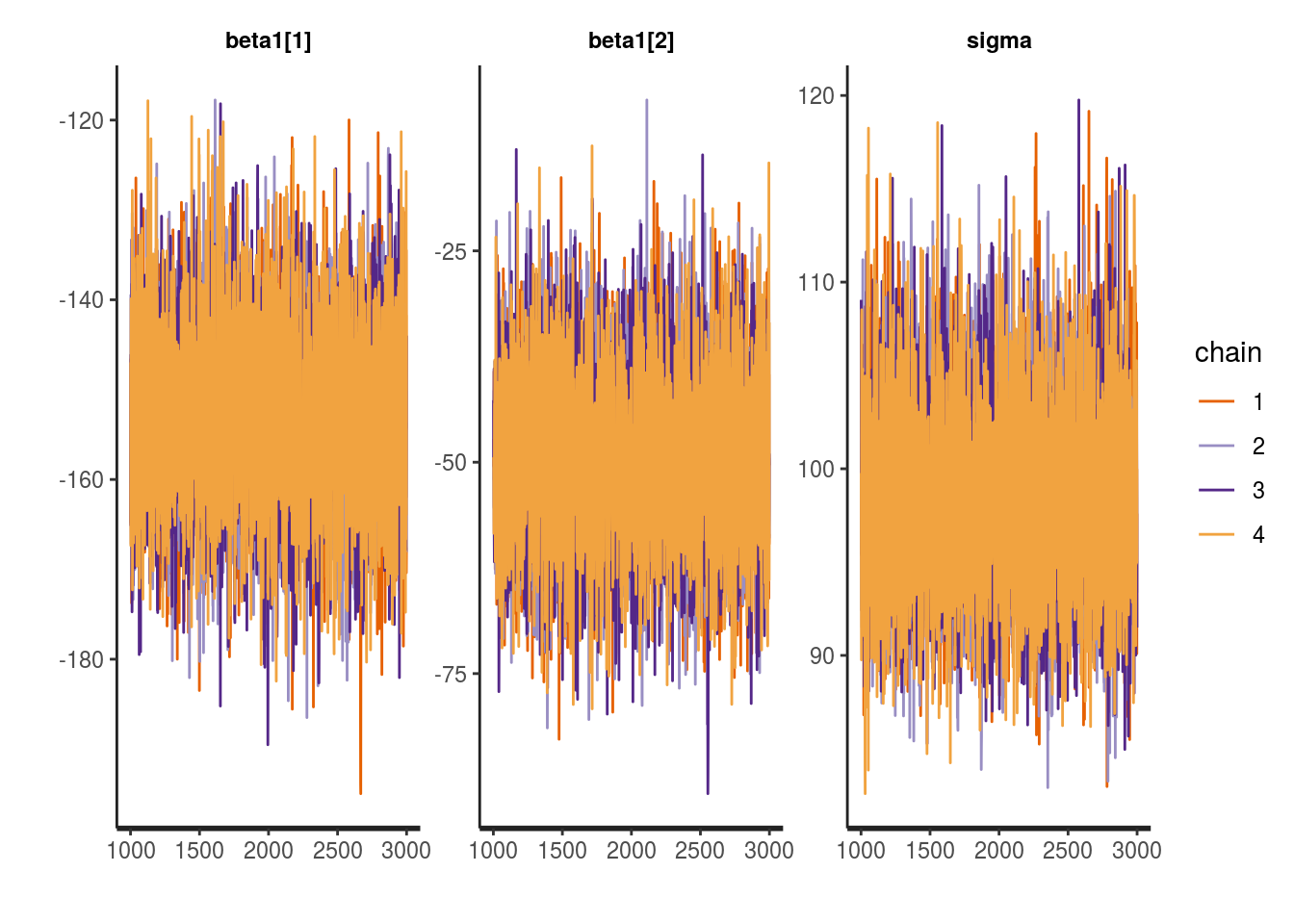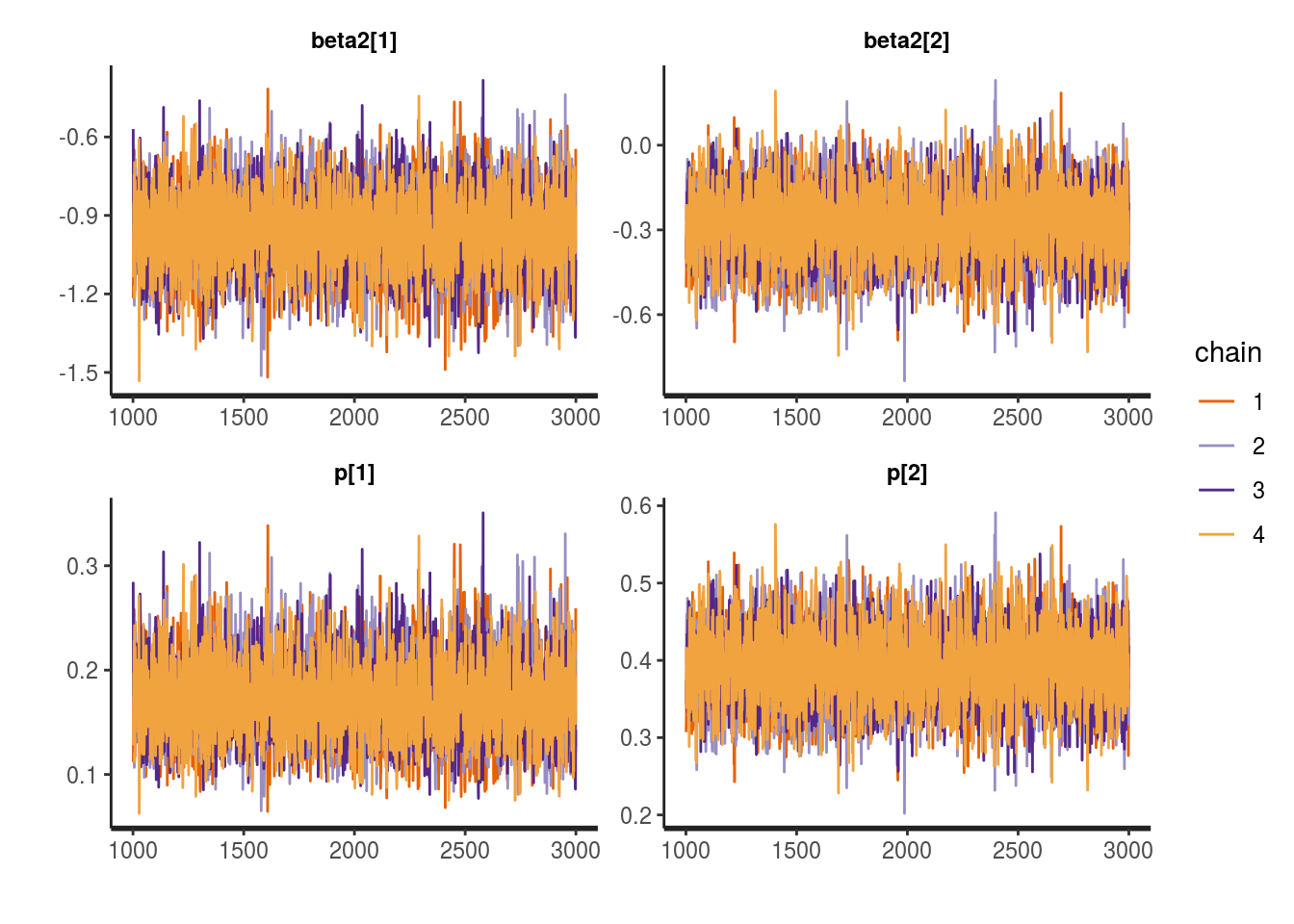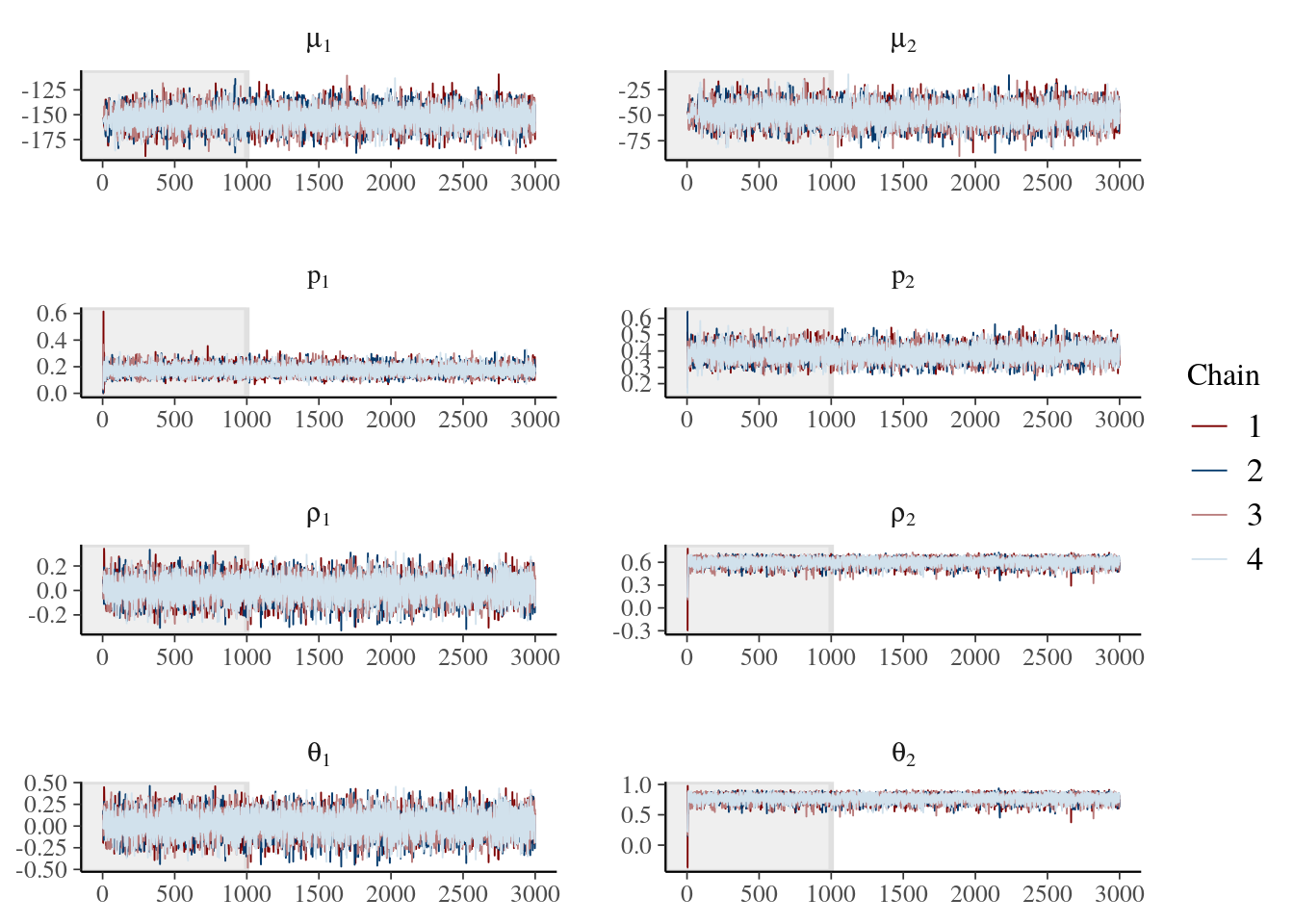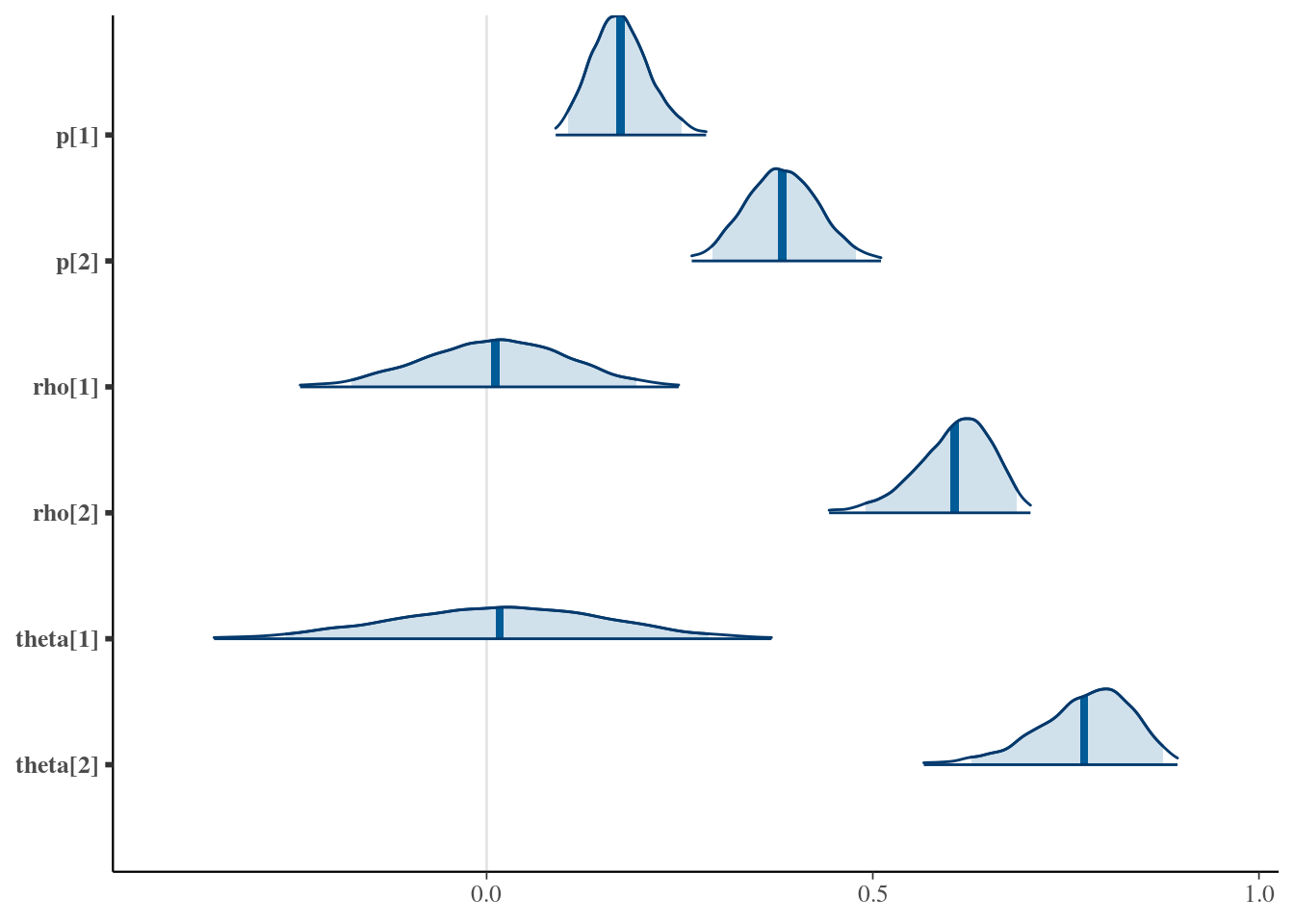# Technical Supplement

• Probability Integral Transformation

Let $$Y$$ be a continuous random variable with df $$F$$ and quantile function $$F^{-1}$$ and define $$F(Y)=Z$$ then $$P(Z \le z) = P(F(Y) \le z)= P(F^{-1}(F(Y)) \le F^{-1}(z)) = P(Y \le F^{-1}(z))=F(F^{-1}(z)) =z$$ which is recognized as the df of a standard uniform random variable. So $$Z \sim Unif(0,1)$$.

• Formula for C-volume with arbitrary $$d$$
$\begin{equation} \Delta_{(\mathbf{a},\mathbf{b}]}C=\sum_{i \in \{0,1\}^d} (-1)^{\sum_{j=1}^d i_j}C(a_{1}^{i_1}b_{1}^{1-i_1},\ldots,a_{d}^{i_d}b_{d}^{1-i_d}) \end{equation}$

where the sum is over the set of $$2^d$$ vectors of size $$d$$ with elements 0 or 1. For $$d=2$$, the set is $$\{(i_1=0,i_2=0),(i_1=0,i_2=1),(i_1=1,i_2=0),(i_1=1,i_2=1)\}$$ and the C-volume is $$\Delta_{(\mathbf{a},\mathbf{b}]}C=C(b_1,b_2)-C(b_1,a_2)-C(a_1,b_2)+C(a_1,a_2)$$. For $$d=3$$ the set is $$\{(i_1=0,i_2=0,i_3=0),(i_1=0,i_2=0,i_3=1),(i_1=0,i_2=1,i_3=0),(i_1=0,i_2=1,i_3=1),(i_1=1,i_2=0,i_3=0),(i_1=1,i_2=0,i_3=1),(i_1=1,i_2=1,i_3=0),(i_1=1,i_2=1,i_3=1)\}$$ and the C-volume is $$\Delta_{(\mathbf{a},\mathbf{b}]}C= C(b_1,b_2,b_3)- C(b_1,b_2,a_3)- C(b_1,a_2,b_3)+ C(b_1,a_2,a_3)- C(a_1,b_2,b_3)+ C(a_1,b_2,a_3)+ C(a_1,a_2,b_3)- C(a_1,a_2,a_3)$$.

• Likelihood for discrete margins
$\begin{equation} \ell(\mathbf{\gamma}_1,\ldots,\mathbf{\gamma}_d,\mathbf{\theta}) = \sum_{i=1}^{n} \log \{ \sum_{(\diamond_1 \cdots \diamond_d) \in \{-,+\}^d } (-1)^{\#\{\diamond_j=-\}} C_{\theta}(F_1(y_{i1}^{\diamond_1};\mathbf{\gamma}_1),\ldots,F_d(y_{id}^{\diamond_d};\mathbf{\gamma}_d)) \} \tag{8} \end{equation}$ Where $$y_{ij}^{+}$$ (with $$\diamond_j=+$$) and $$y_{ij}^{-}$$ (with $$\diamond_j=-$$) refer to evaluation as right limits and left limits, respectively. For $$d=2$$ the innermost sum is over the set $$(\diamond_1,\diamond_2) \in \{-,+\}^2$$, i.e. $$\{(\diamond_1=-,\diamond_2=-),(\diamond_1=-,\diamond_2=+),(\diamond_1=+,\diamond_2=-),(\diamond_1=+,\diamond_2=+) \}$$. So the inner sum $$Q$$ is: $\begin{eqnarray} Q & = &(-1)^{2} C_{\theta}(F_1(y_{i1}^{-};\mathbf{\gamma}_1),F_d(y_{i2}^{-};\mathbf{\gamma}_2)) + (-1)^{1} C_{\theta}(F_1(y_{i1}^{-};\mathbf{\gamma}_1),F_d(y_{i2}^{+};\mathbf{\gamma}_2)) + \nonumber \\ & & (-1)^{1} C_{\theta}(F_1(y_{i1}^{+};\mathbf{\gamma}_1),F_d(y_{i2}^{-};\mathbf{\gamma}_2)) + (-1)^{0} C_{\theta}(F_1(y_{i1}^{+};\mathbf{\gamma}_1),F_d(y_{i2}^{+};\mathbf{\gamma}_2))\nonumber \\ & = & C_{\theta}(F_1(y_{i1}^{-};\mathbf{\gamma}_1),F_d(y_{i2}^{-};\mathbf{\gamma}_2)) - C_{\theta}(F_1(y_{i1}^{-};\mathbf{\gamma}_1),F_d(y_{i2}^{+};\mathbf{\gamma}_2)) - \nonumber \\ & & C_{\theta}(F_1(y_{i1}^{+};\mathbf{\gamma}_1),F_d(y_{i2}^{-};\mathbf{\gamma}_2)) + C_{\theta}(F_1(y_{i1}^{+};\mathbf{\gamma}_1),F_d(y_{i2}^{+};\mathbf{\gamma}_2)) \nonumber \end{eqnarray}$
• Likelihood for mixed margins
Let $$S_1$$ be the set of indices for continuous variables and $$S_2$$ be indices for discrete variables. $\begin{eqnarray} \ell(\mathbf{\gamma}_1,\ldots,\mathbf{\gamma}_d,\mathbf{\theta}) & = & \sum_{i=1}^{n} \{\log c_{\theta,S_1}(F_j(y_{ij};\mathbf{\gamma}_j): j \in S_1) + \sum_{j \in S_1} \log f_j(y_{ij};\mathbf{\gamma}_j) \tag{9} \\ & + & \log \sum_{\diamond_k \in \{-,+\}: k \in S_2} (-1)^{\#\{\diamond_k=-\}} C_{\theta,S_2|S_1}(F_k(y_{ik}^{\diamond_k};\mathbf{\gamma}_k): k \in S_2 |F_j(y_{ij};\mathbf{\gamma}_j) : j \in S_1) \} \nonumber \end{eqnarray}$

Where $$c_{\theta,S_1}$$ is the copula density for the continuous variables (with the discrete margins marginalized out) and $$C_{\theta,S_2|S_1}$$ is the copula for the discrete margins conditional on the continuous margins. The first line of equation (9) is similar to the log-likelihood equation for continuous variables in (5), but uses a copula density where the discrete margins have been averaged out. The second line of equation (9) is similar to the finite differencing for discrete variables in (8) with the modification that the copula is conditioned on the continuous margins.

In addition to the discrete and mixed cases shown above, Joe  also provides log-likelihoods for margins with right-censoring and missing-at-random values. Gunawan et al.  develop extensions for the case when an individual margin has both a discrete and continuous component.

• Mixed continuous/binary margins likelihood from Benefit-Risk example
For $$d=2$$, with the first variable continuous and the second variable discrete the form of the log-likelihood from equation (9) is: $\begin{eqnarray} \ell(\mathbf{\gamma}_1,\mathbf{\gamma}_2,\mathbf{\theta}) & = & \sum_{i=1}^{n} \{ \log c_{\theta,1}(F_1(y_{i1};\mathbf{\gamma}_1)) + \log f_1(y_{i1};\mathbf{\gamma}_1) + \nonumber \\ & & \log \{ (-1)^{1} C_{\theta,2|1}(F_2(y_{i2}^{-};\mathbf{\gamma}_2)|F_1(y_{i1};\mathbf{\gamma}_1)) + (-1)^{0} C_{\theta,2|1}(F_2(y_{i2}^{+};\mathbf{\gamma}_2)|F_1(y_{i1};\mathbf{\gamma}_1)) \} \}\\ & = & \sum_{i=1}^{n} \{ 0 + \log f_1(y_{i1};\mathbf{\gamma}_1) + \nonumber \\ & & \log \{ C_{\theta,2|1}(F_2(y_{i2}^{+};\mathbf{\gamma}_2)|F_1(y_{i1};\mathbf{\gamma}_1)) - C_{\theta,2|1}(F_2(y_{i2}^{-};\mathbf{\gamma}_2)|F_1(y_{i1};\mathbf{\gamma}_1)) \} \} \end{eqnarray}$ Where the first term is 0 since $$C_{\theta,1}(u_1)=\lim_{u_2 \to 1} C_{\theta}(u_1,u_2)=u_1$$ and $$c_{\theta,1}(u_1) = \frac{\partial C_{\theta,1}(u_1)}{\partial u_1} = \frac{\partial}{\partial u_1} u_1=1$$ so $$\log c_{\theta,1}(u_1)=\log 1=0$$. Making the appropriate substitutions and simplifications Costa and Drury show this can be written as: $\begin{eqnarray*} \ell_i(\mu_i,\sigma_i,p_i,\theta) & = & (1-y_{i2}) \log\left(f_1(y_{i1}|\mu_i,\sigma_i) \cdot \Phi \left(\frac{\Phi^{-1}[1-p_i]-\theta_t\cdot\Phi^{-1}[F_1(y_{i1}|\mu_i,\sigma_i)] }{\sqrt{1-\theta_t^2}} \right) \right) \nonumber \\ & & \times y_{i2} \log \left(f_1(y_{i1}|\mu_i,\sigma_i) \cdot \left\{1-\Phi \left(\frac{\Phi^{-1}[1-p_i]-\theta_t\cdot\Phi^{-1}[F_1(y_{i1}|\mu_i,\sigma_i)] }{\sqrt{1-\theta_t^2}} \right) \right\} \right) \end{eqnarray*}$
• Benefit-Risk MCMC model diagnostics
## marginal model diagnostics
#http://mc-stan.org/bayesplot/

print(fit0a, probs=c(0.025, 0.975))
## Inference for Stan model: 968550e09f4560174cecbd127bc5bb9b.
## 4 chains, each with iter=3000; warmup=1000; thin=1;
## post-warmup draws per chain=2000, total post-warmup draws=8000.
##
##              mean se_mean    sd     2.5%    97.5% n_eff Rhat
## beta1  -153.00    0.11  9.77  -171.97  -133.92  8000    1
## beta1   -49.34    0.11 10.05   -68.81   -29.60  8000    1
## sigma       98.41    0.06  5.01    89.22   108.95  7838    1
## lp__     -1017.61    0.02  1.25 -1020.87 -1016.20  4033    1
##
## Samples were drawn using NUTS(diag_e) at Mon Feb 25 17:33:03 2019.
## For each parameter, n_eff is a crude measure of effective sample size,
## and Rhat is the potential scale reduction factor on split chains (at
## convergence, Rhat=1).
color_scheme_set("viridis")
stan_trace(fit0a)print(fit0b, probs=c(0.025, 0.975))
## Inference for Stan model: 14df10564cc86d04063140f36084b07d.
## 4 chains, each with iter=3000; warmup=1000; thin=1;
## post-warmup draws per chain=2000, total post-warmup draws=8000.
##
##             mean se_mean   sd    2.5%   97.5% n_eff Rhat
## beta2   -0.96    0.00 0.15   -1.26   -0.67  6695    1
## beta2   -0.28    0.00 0.13   -0.53   -0.03  7374    1
## p        0.17    0.00 0.04    0.10    0.25  6547    1
## p        0.39    0.00 0.05    0.30    0.49  7374    1
## lp__     -113.47    0.02 1.02 -116.14 -112.49  3453    1
##
## Samples were drawn using NUTS(diag_e) at Mon Feb 25 17:33:58 2019.
## For each parameter, n_eff is a crude measure of effective sample size,
## and Rhat is the potential scale reduction factor on split chains (at
## convergence, Rhat=1).
stan_trace(fit0b)## joint model diagnostics
print(br_fit, probs=c(0.025, 0.975))
## Inference for Stan model: 35135559ebf8c381d1a6ad94ea441ba4.
## 4 chains, each with iter=3000; warmup=1000; thin=1;
## post-warmup draws per chain=2000, total post-warmup draws=8000.
##
##              mean se_mean    sd     2.5%    97.5% n_eff Rhat
## beta1  -152.97    0.11 10.17  -172.84  -133.05  8000    1
## beta1   -49.60    0.13  9.88   -68.88   -30.33  5976    1
## s        99.68    0.08  7.28    86.58   115.08  8000    1
## s        97.69    0.08  6.88    85.07   112.14  8000    1
## beta2    -0.95    0.00  0.15    -1.25    -0.67  8000    1
## beta2    -0.30    0.00  0.13    -0.55    -0.05  5464    1
## omega     0.02    0.00  0.14    -0.26     0.29  8000    1
## omega     0.77    0.00  0.06     0.63     0.88  7168    1
## mu     -152.97    0.11 10.17  -172.84  -133.05  8000    1
## mu      -49.60    0.13  9.88   -68.88   -30.33  5976    1
## p         0.17    0.00  0.04     0.11     0.25  8000    1
## p         0.38    0.00  0.05     0.29     0.48  5486    1
## theta     0.02    0.00  0.14    -0.26     0.29  8000    1
## theta     0.77    0.00  0.06     0.63     0.88  7168    1
## rho       0.01    0.00  0.09    -0.17     0.19  8000    1
## rho       0.61    0.00  0.05     0.49     0.69  7066    1
## lp__     -1294.09    0.03  2.02 -1298.88 -1291.16  3835    1
##
## Samples were drawn using NUTS(diag_e) at Mon Feb 25 17:35:10 2019.
## For each parameter, n_eff is a crude measure of effective sample size,
## and Rhat is the potential scale reduction factor on split chains (at
## convergence, Rhat=1).
br_posterior <- extract(br_fit, inc_warmup = TRUE, permuted = FALSE)

color_scheme_set("mix-blue-red")
mcmc_trace(br_posterior, pars = c("mu", "mu", "p", "p", "rho", "rho",
"theta", "theta"), n_warmup = 1000,
facet_args = list(nrow = 4, labeller = label_parsed))br_posterior2 <- extract(br_fit, inc_warmup = FALSE, permuted = FALSE)

mcmc_areas(br_posterior2, pars = c("mu", "mu"),
prob = 0.95, prob_outer = 0.99, point_est = "mean")mcmc_areas(br_posterior2, pars = c("p", "p", "rho", "rho",
"theta", "theta"),
prob = 0.95, prob_outer = 0.99, point_est = "mean")### References

13. Joe H. Dependence modeling with copulas. Boca Raton: CRC Press, Taylor & Francis Group; 2015.

70. Gunawan D, Khaled MA, Kohn R. Mixed marginal copula modeling. Journal of Business & Economic Statistics [Internet] 2018 [cited 2018 Oct 12];1–11. Available from: https://www.tandfonline.com/doi/full/10.1080/07350015.2018.1469998